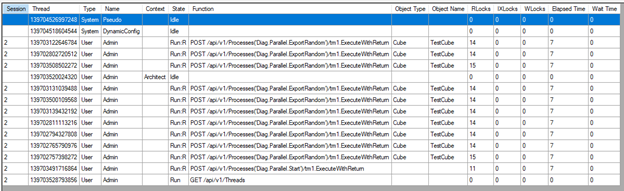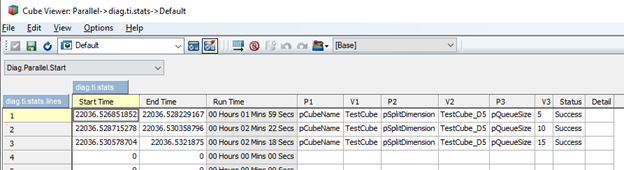# Planning Analytics

View Only

### Parallel Processing with Turbo Integrator

#### By STUART KING posted Tue May 05, 2020 03:37 PM

Parallel Processing with Turbo Integrator

TM1 Server takes advantage of multi-threaded processors in several ways.  In the TM1 10.2.2 release, IBM introduced the Multi-Threaded Query (MTQ) feature.  MTQ allows views to be calculated using multiple threads.  Before MTQ each view would only use a single thread to determine rule derived and consolidated cell values.  MTQ can greatly reduce the time it can take for TM1 to work its magic.  The MTQ feature is also applied to TI processes when a view is used as the data source.

Processing a cube view as a data source is only one area of processing in a TI process.  What if we want to perform calculations in the TI script, or load data into a cube using multiple threads?  In these cases, the solution may be to divide the work into many TI processes that can be run in parallel.

Two Important Things to Consider

1 – How to Divide Work into Separate TI Processes

In the provided TM1 model we will process leaf level data in a cube.  The cube being used as a data source is sliced into views that are uniquely defined using leaf elements in one dimension.  Each of these unique views will be used as a data source by an instance of the same TI process.

In a real world use case we may consider using a dimension that represents months, cost centers, or some other logical way to divided up the total workload.  The dimension being used to divide the cube into views is out ‘split dimension’.  The number of views to be processed will be the total number of TI processes to be executed.

2 – How Many Processes to run in Parallel

Determine the correct number of TI processes to run in parallel.  This number is likely much smaller than the total number of TI processes to be executed (the total number of views based on the split dimension).  The number of processes to run in parallel should mostly depend on the number of cores on the server running TM1.  In general, the more cores on the server, the more TI processes you can run in parallel.

Let’s think about a few possible situations.  Assume the split dimension gives us 20 views and the server running TM1 has 24 cores.  In this case all 20 views can be processed in separate TI processes instances in parallel.  Assuming no other significant CPU activity in the TM1 database or server we would expect to see that 20/24 cores actively being used.

What about the case where the split dimension creates 200 views and the server only has 24 cores.  Running all 202 processes in parallel would surely overload the server.  The net result of completing all processes would likely be sub-optimal.  In this case, optimal throughput would likely happen when we run 24 processes in parallel (one process per core) until all 200 are complete.   We need a way to constrain the number of processes running in parallel to be lower than the total number of processes.  We need a queue!  In the attached example we use a parent TI process to manage the workload using a queue.  The parent process is will start child processes that do the work, process views created by the split dimension.  The queue is used to track which processes are currently running.  The parent process also needs a mechanism to detect when child processes are complete and start additional child processes.

Let’s examine each of these components of our parent process:

The Queue

In this example the queue is build using a simple string variable.  The queue variable contains a list of the element names that are used to define the views currently being processed by TI.  Note that this queue is not first come first serve since we do not know which of the currently running TI processes might finish first.

Detecting if a Child TI Process is Complete

When one of the parallel TI process completes, we must remove that process from the queue, and add another process to the queue.  Each of the child TI processes creates a file as the final step in the TI process (last line of the epilog section).  This file is used as the signal that the TI process is complete and ready to be removed from the queue.   Having the TI process write a flag value to a cube and checking for that flag will not work!  Remember that when a TI process is executing it cannot see changes to cells that happen after the TI started (unless the change was made by that TI process).

The parent TI process makes use of the FileExists TI function to check for the file created by the child TI process.  When this file is found a TI process is removed from the queue.  The next child TI process is started assuming any child TI processes remain to be run.

Starting a Child TI Process

Starting another TI process on its own thread had traditionally been accomplished using the ExecuteCommand function to call the TM1RunTI command line.  Recent versions of TM1 Server include a new TI function named RunProcess that can also start a TI process running in its own thread.  A major advantage to using RunProcess over TM1RunTI is that an additional login is not required by RunProcess.  Avoiding one more login reduces the chance for lock contention.  RunProcess is also much easier to implement and more robust.

Using the Sample TM1 Model

How the Sample TM1 Model Works

The attached TM1 model includes a cube named TestCube that can be populated with random values using parallel TI processes.  This model emulates both exporting data from a cube and importing data into a cube with parallel processes.  Real world examples may also include some transformation of the data with TI script.  A Linux and Windows version of the TM1 model is included,

Before running any of the TI processes be sure to examine the diag.control.cube cube.  This cube contains string values that are used to determine the directory where flat files are exported and where the flag files are created.If running on Windows be sure to set the platform value to WIN.  On Linux the platform value should be set to IX.  This parameter is used to determine the correct slash character to use in file paths.  The other important parameters are export directory and queue directory.  Make sure the export directory and queue directory exist before testing any of the TI processes.

The Diag.Parallel.Start TI Process

The TI process used to start the overall workload is named Diag.Parallel.Start.  A few notes about the parameters for this process:

pCubeName – The name of the cube you want to populate.  In this case we are going populate the cube named TestCube.

pSplitDimension – The split dimension.  Leaf elements in this dimension are used to divide the workload.  The number of leaf elements in this dimension will be the total number of child processes that will be executed.

pQueueSize – The maximum number of child TI processes that will run in parallel.Given the values in the above screenshot, and assuming the TestCube_D5 dimension has 100 leaf elements, we can expect that a total of 100 processes will be run, with a maximum of 11 processes running at any given time, the parent process plus 10 instances of the child process.

When the Diag.Parallel.Start process is run expect to see the following thread activity.The Diag.Parallel.ExportRandom creates a random value for each intersection in the view it’s processing.  These random values are exported to a flat file.  Once all intersections in the view are processed the Diag.Parallel.ExportRandom process then calls the Diag.Parallel.Import process using the ExcuteProcess TI function.  Diag.Parallel.Import imports values from the flat file into TestCube.   Before Diag.Parallel.Import completes, it creates the file that is used to signal the child process is complete.

Tracking Results

The attached TM1 model may be used to determine the optimal queue size.  Remember that the optimal queue size will depend on the core count of the server running TM1.  Since Diag.Parallel.Start runs for the total length of all child processes we only need to measure the runtime of Diag.Parallel.Start.  Diag.Parallel.Start can be run with different queue sizes to determine which queue size is optimal (lowest time to complete Diag.Parallel.Start).  A good starting point for the queue size is the core count of the server running TM1.

Runtime of Diag.Parallel.Start is tracked in the cube named diag.ti.stat.  This cube contains a view that tracks parameter values and total runtime of each execution of the Diag.Parallel.Start process.  The following screenshot shows a comparison of three runs using the same cube and split dimension, but with different queue sizes (5, 10 and 15) for each execution.Testing with Larger Cubes

It may also be necessary to repeat the test with a cubes and dimensions of different sizes.  A cube with more dimensions, or more elements in some dimensions may better emulate a real-world use case.  The sample model includes a process named Diag.Model.CubeCreate that can be used to generate cubes to test with.Diag.Model.CubeCreate can be used to generate cubes with up to 17 dimensions.   You can specify the number of dimensions and the number of leaf elements in each dimension.  The names of the dimensions and their elements are generated automatically.  Here is a description of the parameters used in this process:

pCubeName – The name of the cube to be created or recreated

pDimensionCount – The number of dimensions in the cube.  Max of 17 dimensions.  Dimension names are generated automatically.

pDimensionSizes – The number of leaf level elements in each of the dimensions.  In the example above the first and seconds dimension have 10 elements, the 3rd dimension will have 20 leaf elements, and the 4rth and 5th dimension will each have 100 leaf members.  Consolidations are generated automatically.

pElementNameSize – The string length of the element names.  A typically value of 8 should work for most dimensions below 10000 leaf elements.

pRecreateCube – Setting this to Y will delete and recreate an existing cube and the dimension used in that cube.

Attached TM1 Model Files